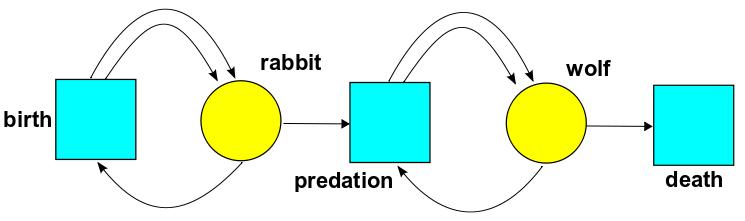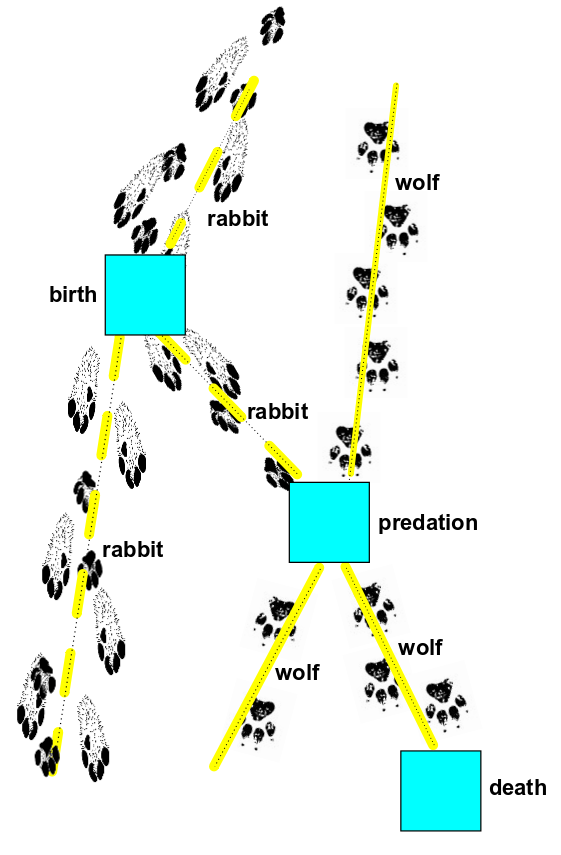# The Azimuth Project Blog - network theory (part 2)

This page is a blog article in progress, written by John Baez. For the final polished version, go to the Azimuth Blog.

Today I’d like to start telling you about some research Jacob Biamonte and I are doing on stochastic Petri nets, quantum field theory, and category theory. It’ll take a few blog entries to cover this story, which is part of a larger story about network theory.

Stochastic Petri nets are one of many different diagrammatic languages people have evolved to study complex systems. We’ll see how they’re used in chemistry, molecular biology, population biology and queuing theory, which is roughly the science of waiting in line. If you’re a faithful reader of this blog, you’ve already seen an example of a Petri net taken from chemistry:It shows some chemicals and some reactions involving these chemicals. To make it into a stochastic Petri net, we’d just label each reaction by a nonnegative real number: the reaction rate constant, or rate constant for short.

In general, a Petri net will have a set of states, which we’ll draw as yellow circles, and a set of transitions, which we’ll draw as blue rectangles. Here’s a Petri net from population biology:Now, instead of different chemicals, the states are different species. And instead of chemical reactions, the transitions are processes involving our species.

This Petri net has two states: rabbit and wolf. It has three processes:

• In birth, one rabbit comes in and two go out. This is a caricature of reality: these bunnies reproduce asexually, splitting in two like amoebas.

• In predation, one wolf and one rabbit come in and two wolves go out. This is a caricature of how predators need to eat prey to reproduce.

• In death, one wolf comes in and nothing goes out. Note that we are pretending rabbits don’t die unless they’re eaten by wolves.

If we labelled each transition with a rate constant, we’d have a stochastic Petri net.

To make this Petri net more realistic, we’d have to make it more complicated. Nonetheless, it already leads to an interesting model of population dynamics: a special case of the so-called Lotka-Volterra predator-prey model. We’ll see the details soon.

More to the point, this Petri net illustrates some possibilities that our previous example neglected. Every transition has some ‘input’ states and some ‘output’ states. But a state can show up more than once as the output (or input) of some transition. And as we see in ‘death’, we can have a transition with no outputs (or inputs) at all.

But let me stop beating around the bush, and give you the formal definitions. They’re simple enough:

Definition - A Petri net consists of a set $S$ of states and a set $T$ of transitions, together with a function

$i : S \times T \to \mathbb{N}$

saying how many copies of each state shows up as input for each transition, and a function

$o: S \times T \to \mathbb{N}$

saying how many times it shows up as output.

Definition - A stochastic Petri net is a Petri net together with a function

$r: T \to [0,\infty)$

giving a rate constant for each transition.

Starting from any stochastic Petri net, we can get two things. First:

• The master equation. This says how the probability that we have a given number of things in each state changes with time.

Since stochastic means ‘random’, the master equation is what gives stochastic Petri nets their name. This is the main thing I’ll be talking about in future blog entries. But not right away!

Why not?

In chemistry, we typically have a huge number of things in each state. For example, a gram of water contains about $3 \times 10^{22}$ water molecules, and a smaller but still enormous number of hydroxide ions (OH-), hydronium ions (H3O+), and other scarier things. These things blunder around randomly, bump into each other, and sometimes react and turn into other things. There’s a stochastic Petri net describing all this, as we’ll eventually see. But in this situation, we don’t want to know the probability that there are, say, exactly $310,1849,578,476,264$ hydronium ions. That would be too much information! We’d be quite content knowing the expected value of the number of hydronium ions, so we’d be delighted to have a differential equation describing how this changes with time.

And luckily, such an equation exists—and it’s much simpler than the master equation. So, today we’ll talk about:

• The rate equation. This says how the expected number of things in each state changes with time.

But first, I hope you get the overall idea. The master equation is stochastic: at each time the number of things in each state is a random variable taking values in $\mathbb{N}$, the set of natural numbers. The rate equation is deterministic: at each time the expected number of things in each state is a non-random variable taking values in $[0,\infty)$, the set of nonnegative real numbers. If the master equation is the true story, the rate equation is only approximately true—but the approximation becomes good in some limit where the expected value of the number of things in each state is large, and the standard deviation is comparatively small.

If you’ve studied physics, this should remind you of other things. The master equation should remind you of the quantum harmonic oscillator, where energy levels are discrete, and probabilities are involved. The rate equation should remind you of the classical harmonic oscillator, where energy levels are continuous, and everything is deterministic.

When we get to the ‘original research’ part of our story, we’ll see this analogy is fairly precise! We’ll take a bunch of ideas from quantum mechanics and quantum field theory, and tweak them a bit, and show how we can use them to describe the master equation for a stochastic Petri net.

Indeed, the random processes that the master equation describes can be drawn as pictures:This looks like a Feynman diagram, with animals instead of particles! It’s pretty funny, but the resemblance is no joke: the math will back it up.

I’m dying to explain all the details. But just as classical field theory is easier than quantum field theory, the rate equation is simpler than the master equation. So we should start there.

## The rate equation

If you hand me a stochastic Petri net, I can write down its rate equation. Instead of telling you the general rule, which sounds rather complicated at first, let me do an example. Take the Petri net we were just looking at:We can make it into a stochastic Petri net by choosing a number for each transition:

• the birth rate constant $\beta$

• the predation rate constant $\gamma$

• the death rate constant $\delta$

Let $x(t)$ be the number of rabbits and let $y(t)$ be the number of wolves at time $t$. Then the rate equation looks like this:

$\frac{d x}{d t} = \beta x - \gamma x y$
$\frac{d y}{d t} = \gamma x y - \delta y$

It’s really a system of equations, but I’ll call the whole thing “the rate equation” because later we may get smart and write it as a single equation.

See how it works?

• We get a term $\beta x$ in the equation for rabbits, because rabbits are born at a rate equal to the number of rabbits times the birth rate constant $\beta$.

• We get a term $- \delta y$ in the equation for wolves, because wolves die at a rate equal to the number of wolves times the death rate constant $\delta$.

• We get a term $- \gamma x y$ in the equation for rabbits, because rabbits die at a rate equal to the number of rabbits times the number of wolves times the predation rate constant $\gamma$.

• We also get a term $\gamma x y$ in the equation for wolves, because wolves are born at a rate equal to the number of rabbits times the number of wolves times $\gamma$.

Of course I’m not claiming that this rate equation makes any sense biologically! For example, think about predation. The $\gamma x y$ terms in the above equation would make sense if rabbits and wolves roamed around randomly, and whenever a wolf and a rabbit came within a certain distance, the wolf had a certain probability of eating the rabbit and giving birth to another wolf. At least it would be make sense in the limit of large numbers of rabbits and wolves, where we can treat $x$ and $y$ as varying continuously rather than discretely. That’s a reasonable approximation to make sometimes. Unfortunately, rabbits and wolves don’t roam around randomly, and wolf doesn’t spit out a new wolf each time it eats a rabbit.

Despite that, the equations

$\frac{d x}{d t} = \beta x - \gamma x y$
$\frac{d y}{d t} = \gamma x y - \delta y$

are actually studied in population biology. As I said, they’re a special case of the Lotka-Volterra predator-prey model, which looks like this:

$\frac{d x}{d t} = \beta x - \gamma x y$
$\frac{d y}{d t} = \epsilon x y - \delta y$

The point is that while these models are hideously oversimplified and thus quantitatively inaccurate, they exhibit interesting qualititative behavior that’s fairly robust. Depending on the rate constants, these equations can show either a stable equilibrium or stable periodic behavior. And we go from one regime to another, we see a kind of catastrophe called a “Hopf bifurcation”. I explained all this in week308 and week309. There I was looking at some other equations, not the Lotka-Volterra equations. But their qualitative behavior is the same!

If you want stochastic Petri nets that give quantitatively accurate models, it’s better to retreat to chemistry. Compared to animals, molecules come a lot closer to roaming around randomly and having a chance of reacting when they come within a certain distance. So in chemistry, rate equations can be used to make accurate predictions.

But I’m digressing. I should be explaining the general recipe for getting a rate equation from a stochastic Petri net! You probably can’t guess it from just one example. So, next time I’ll do more examples, and maybe even write down a general formula for how it works. But for now, you can try this:

Puzzle - Can you write down a stochastic Petri net whose rate equation is the Lotka-Volterra predator-prey model:

$\frac{d x}{d t} = \beta x - \gamma x y$
$\frac{d y}{d t} = \epsilon x y - \delta y$

for arbitrary $\beta, \gamma, \delta, \epsilon \ge 0$? If not, for which values of these rate constants can you do it?

If you want to study a bit on your own, here are some great online references on stochastic Petri nets and their rate equations:

I should admit that the first two talk about ‘chemical reaction networks’ instead of Petri nets. That’s no big deal: any chemical reaction network gives a Petri net in a pretty obvious way. You can probably figure out how; if you get stuck, just ask.

Also, I should admit that Petri net people say place where I’m saying state.

Here are some other references, which aren’t free unless you have an online subscription or access to a library:

• Peter J. Haas, Stochastic Petri Nets: Modelling, Stability, Simulation, Springer, Berlin, 2002.

• F. Horn and R. Jackson, General mass action kinetics, Archive for Rational Mechanics and Analysis 47 (1972), 81–116.

• Ina Koch, Petri nets – a mathematical formalism to analyze chemical reaction networks, Molecular Informatics 29 (2010), 838–843.

• Darren James Wilkinson, Stochastic Modelling for Systems Biology, Taylor & Francis, New York, 2006.

category: blog